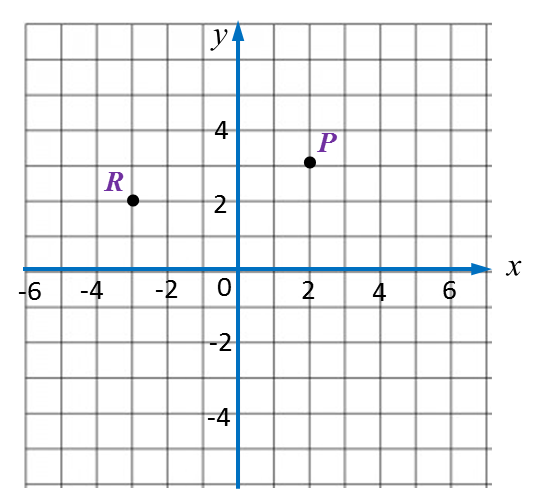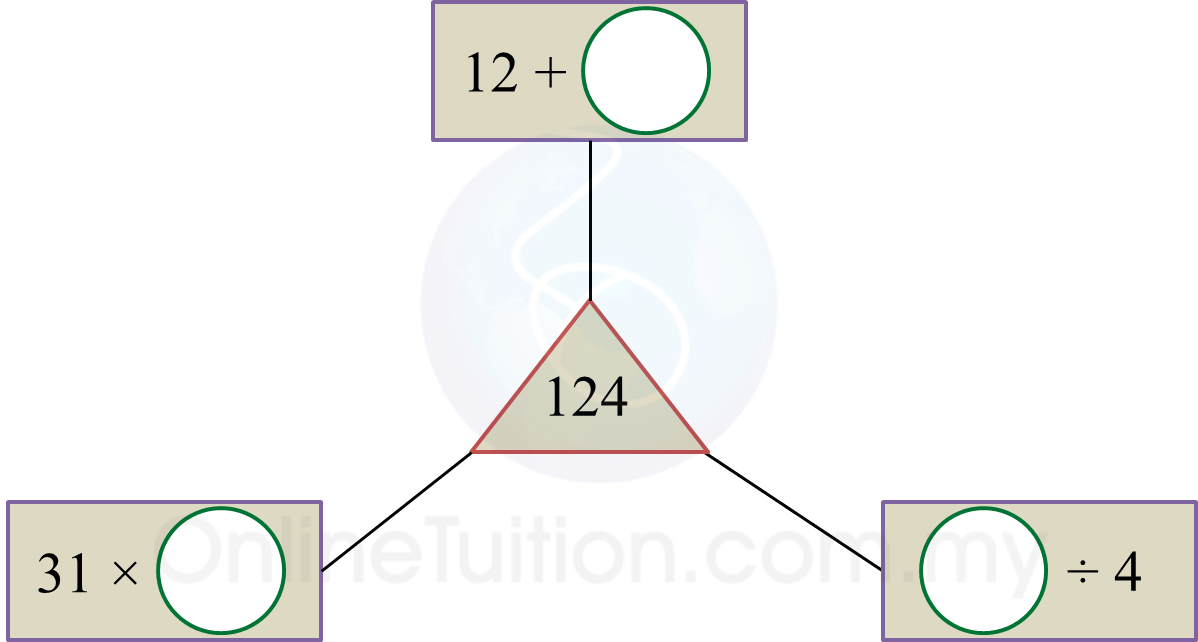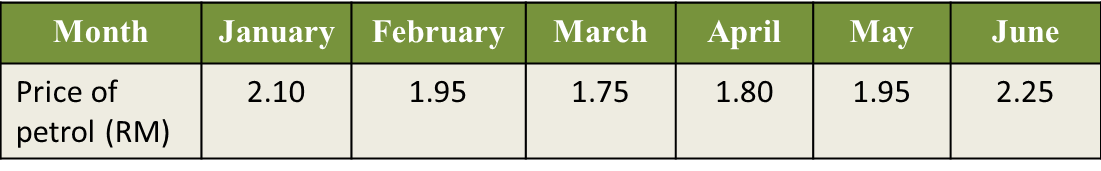# PT3 Mathematics 2017, Question 1

Question 1 (a):
Diagram 1.1 shows a Cartesian plane.(i) State the coordinates of point P.
(ii) On Diagram 1.1, plot Q (4, –2).
(iii) Point R is [   ] units to the left of the y-axis and [   ] units above the x-axis.

Solution:
(i)
P = (2, 3)

(ii)(iii)
Point R is [ 3  ] units to the left of the y-axis and [ 2  ] units above the x-axis.

Question 1 (b):
In Diagram 1.2, fill in each circle with the correct number in order to obtain the value in the triangle shown.Solution:Question 1 (c):
Table 1 shows the price of petrol per litre in a period of 6 months.(i) State the month which the price of petrol is the lowest.
(ii) Ahmad uses 50 litres of petrol for his motorcycle every month.
Calculate the total cost, in RM, for the period of six months.

Solution:
(i)
1.